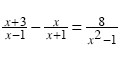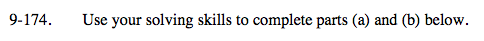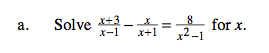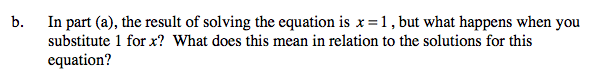Home > A2C > Chapter 9 > Lesson 9.3.3 > Problem9-174

9-174.
1. Use your solving skills to complete parts (a) and (b) below. Homework Help ✎

1. Solvefor x.

2. In part (a), the result of solving the equation is x = 1, but what happens when you substitute 1 for x? What does this mean in relation to the solutions for this equation?Multiply both sides by (x − 1)(x + 1).The equation has no solution.